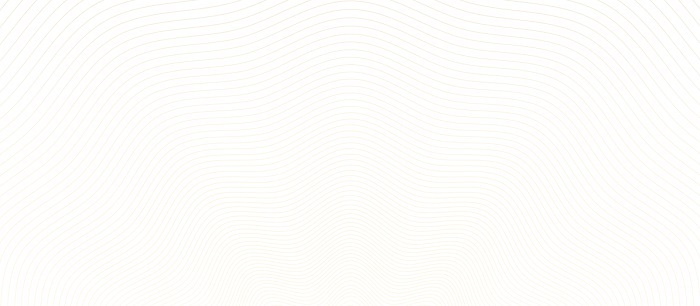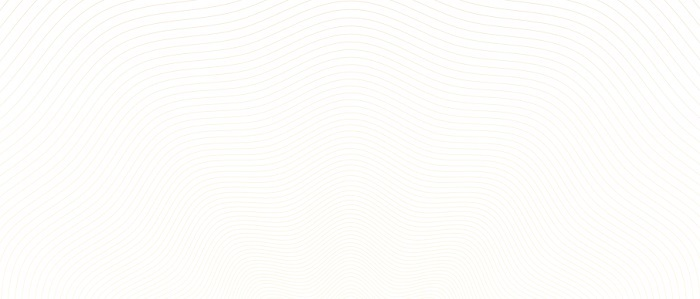# Check if the image is empty using OpenCV Python

OpenCV is an Open Source Computer Vision Library in python. It is one of the image processing libraries in python and it uses the python Numpy library so that all the image arrays are represented as a ndarray type. OpenCV-python needs the numpy library, we need to make sure that the numpy is also installed in our python interpreter.

In this article, we will see the different ways to check if the given image is empty or not using OpenCV Python. Let’s observe the input-output scenarios to understand how to check if the given image is empty or not using Python OpenCV.

## Input Output Scenarios

Assuming we have a blank input image and in the output, we will see a statement saying whether the given image is empty or not.

### Input Image### Output

Image is empty


Let’s discuss what are the different ways to check if the given image is empty or not.

## With unique pixel count

In general, a blank/empty image has more unique pixel values throughout the image. By comparing the unique pixel count with a threshold value, we can identify the empty images.

### Example

In this example, we will use the np.unique() method to get the unique elements.

import numpy as np
import cv2

def is_empty(img):
np.reshape(image, (-1,1))
u, count_unique = np.unique(image, return_counts =True)

if count_unique.size< 10:
return "Image is empty"
else:
return "Image is not empty"

print(is_empty('whh.jpg'))


### Input image### Output

Image is empty


## With standard deviation

The Standard deviation of an empty image should be close to zero. Usually, a blank/empty image may have more uniform pixel values throughout the image. And we can calculate the degree of uniformity using the standard deviation. If the value falls below a certain threshold value, then we can say that the image is blank.

### Example

Let’s take a “blank.png” image and verify it by calculating the standard deviation.

import numpy as np
import cv2

def is_empty(img):
np.reshape(image, (-1,1))
std_dev=np.std(image)

if std_dev < 1:
return "Image is empty"
else:
return "Image is not empty"

print(is_empty('Blank.png'))


### Output

Image is empty


We have used the Numpy.std() method to calculate the standard deviation of image pixel values.

## With summing the pixel values

The pixel values of an empty/black image are zero. If the sum of pixels is not equal to zero then we can say that the given image is not empty. If the sum is equal to zero then the given image is empty.

### Example

Initially, we will create a blank image using the numpy.zeros() method. Then verify if the given image is empty or not.

import numpy as np
import cv2

blank_image2 = np.zeros((100,100,3), dtype=np.uint8)
cv2.imwrite("result.jpg", blank_image2)

def is_empty(img):

# Checking if the image is empty or not
if np.sum(image) == 0:
result = "Image is empty!"
else:
result = "Image is not empty!"
return result

print(is_empty('result.jpg'))


### Output

Image is empty


## Details of The Resultant Image

The result image is created by the cv2.imwrite() method of numpy array data. Then the result.jpg image is given to the defined is_empty() function to check if the given image is empty or not.

Updated on: 30-May-2023

552 Views